# College Chemistry : Le Chatelier's Principle

## Example Questions

### Example Question #1 : Le Chatelier's Principle

Consider the following equation at equilibrium: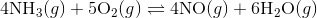What would happen if more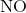were added to the system?

There is not enough information to determine the effects of adding moreinto the system.

There would be no change in the system.

The reaction would shift towards the products.

The reaction would shift towards the reactants.

The reaction would shift towards the reactants.

Explanation:

Recall Le Chatelier's principle: A chemical system at equilibrium will shift in the direction that minimizes the disturbance to the system.

Before the addition of, the system is in equilibrium, meaning the reaction quotient is equal to the equilibrium constant;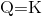.

However, after the addition of, a product, the reaction quotient is now less than the equilibrium constant;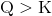.

In order to maintain equilibrium, the reaction will then shift left to favor the reactants in this chemical system.

### Example Question #2 : Le Chatelier's Principle

Consider the following equation at equilibrium: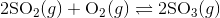What would happen if more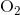were added into the equation?

There would be no change.

Less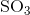would be produced.

More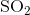would be produced.

Morewould be produced.

Morewould be produced.

Explanation:

Recall Le Chatelier's principle: A chemical system at equilibrium will shift in the direction that minimizes the disturbance to the system.

Before the addition of, the system is in equilibrium, meaning the reaction quotient is equal to the equilibrium constant;.

However, after the addition of, a reactant, the reaction quotient is now less than the equilibrium constant;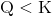.

In order to maintain equilibrium, the reaction will then shift right of favor the formation of more products.

### Example Question #3 : Le Chatelier's Principle

Consider the following exothermic equation: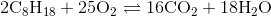Which of the following actions will cause the products to be favored?

Increase the amount of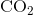.

Decrease the amount of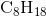.

Decrease the temperature at which the reaction is conducted.

Increase the temperature at which the reaction is conducted.

Decrease the temperature at which the reaction is conducted.

Explanation:

Since the equation is exothermic, you can think of heat as another product of the reaction: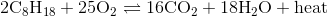Apply LeChatelier's principle to this equation.

If the temperature at which the reaction is decreased, that is akin to decreasing the amount of product made, thus causing the reaction to shift towards the products.